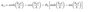# Numerical Integration of Double integrals

ruzfactor
I have a double integral:
∫∫sin^2(∏x/A)*sin^2(∏y/B)dxdy

A=length along x
B=length along y

ranges: 0 to A(for x) & 0 to B (for y)

Analytical result is: A*B/4 (unit^2)

Now, I want to evaulate it numerically using trapezoidal rule. Infact, I have done it but not sure whether it is a right procedure although got the same result as analytical. Here is what I did:

for integrating wrt x I chose y=B and x=0 to A (with an interval Δx)

evaluated the different values of the function for different x values. Then applied the trapezoidal rule using the evaluated values of the function and x. Got a result A/2 (unit).
Similar thing I did only this time x=A and y=0 to B (with Δy interval). Got result B/2 (unit).

Combining this two results gives me the same result as the analytical one. Is this a right way to do numerical integration of a double integral?

Another question that is bugging me is the unit of the result of a numerical integration. It might sound stupid to most of you but this has been confusing me for the last few days.

As we all know integration of a function is the area under the curve of that function. Now if a numerical integration technique is applied on that area, I would get the result of the intergation within its limits. Now what would be the unit this case? unit^2?

Then why the function above if integrated wrt x or y produces a result whose unit is just the unit (e.g. m)? This is where it is confusing. In some examples I have seen, if the unit is not in say m then the whole result becomes inconsistent.

For example
∫ψ(x)dx if integrated analytically in 0 to L range the result is L (m). Same found from numerical integration. If the integration is simply the area under the curve, then the unit should have been m^2 when doing numerically. This is where I have been arguing with someone. I hope someone would explain this stupid argument. Thanks.

Homework Helper
Gold Member
2022 Award
∫ψ(x)dx if integrated analytically in 0 to L range the result is L (m). /QUOTE]
It depends what dimension you think psi has. If consider you are calculating an area then psi also has length dimension, so the integral has dimension length squared.

ruzfactor
Please see the attached for the function psi.

#### Attachments

•psi.jpg
5.6 KB · Views: 370
Homework Helper
Gold Member
2022 Award
Please see the attached for the function psi.
What the function is algebraically is irrelevant. What matters for your question is whether you regard it has having a length dimension. If you do, the integral will have dimension length squared (I'm assuming x has length dimension). If you don't then the integral is not computing an area - it only seems like an area because you drew it as a graph ( thereby giving psi length dimension).

ruzfactor
so the unit would depend on the unit I am considering for the function. Although the integral is not computing an area but the integral is found from the area of the curve drawn from the function along its limits numerically.

e.g. ∫x^3dx= x^4/4 [m^4] (limit say 0 to 5)

So I can evaluate it numerically by plotting a curve and computing the area under the curve within limits. I hope I am not pushing things further! :S

ruzfactor
Is my way of doing the double integration correct using the trapezoidal rule?